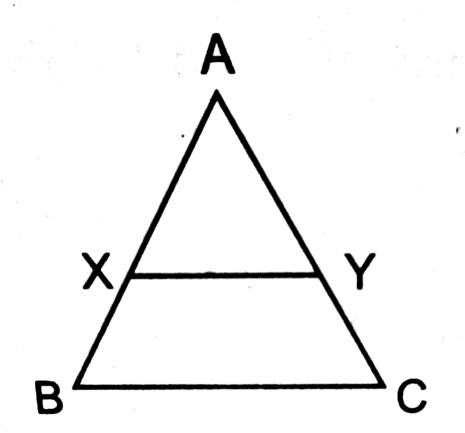# In a triangle ABC, a line XY drawn parallel to BC meeting AB in X and AC in Y. The area of the triangle AXY is half of the area of the triangle ABC. XY divides AB in the ratio of (a) 1:(b):(1) (c) 1: (1) (d)## Question ID - 50090 :- In a triangle ABC, a line XY drawn parallel to BC meeting AB in X and AC in Y. The area of the triangle AXY is half of the area of the triangle ABC. XY divides AB in the ratio of (a) 1:(b):(1) (c) 1: (1) (d)3537So,Let areasq. units.

Area () =sq. units.Next Question :
 The area of two similar triangles are 12 cm2 and 48 cm2. If the height of the smaller one is 2.1 cm, then the corresponding height of the bigger one is (a) 0.525 cm (b) 4.2 cm (c) 4.41 cm (d) 8.4 cm